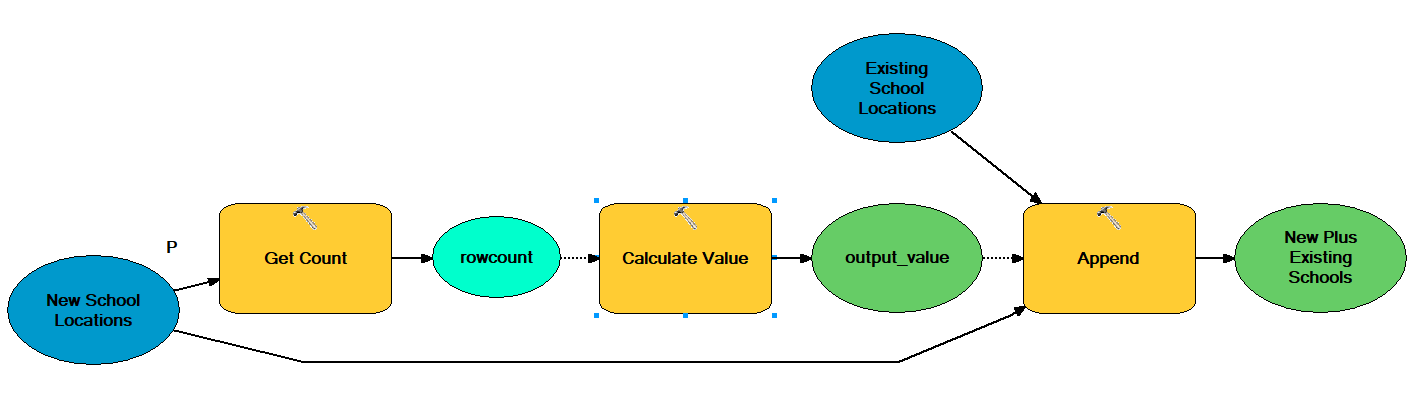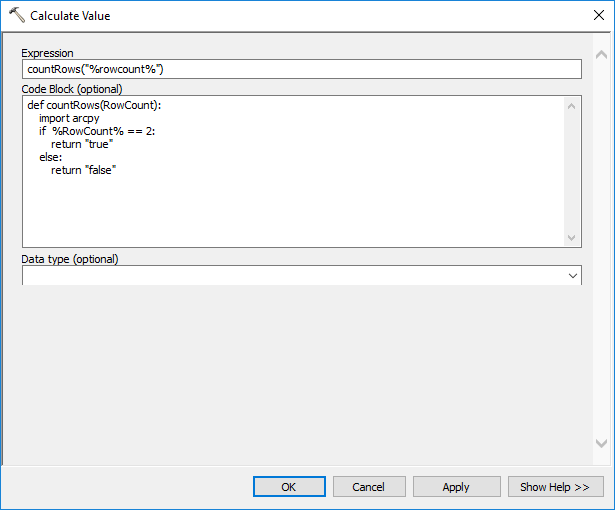HOW TO

# Restrict Feature Creation When Using Feature Sets in ModelBuilder

## Summary

In some cases, it is required to input user-created interactive features when running a model using Feature Sets. The issue is that often the creator of the model wishes to restrict the user from inputting more than the required number of minimum or maximum inputs.

This solution does not impede the user from selecting more than required minimum or maximum inputs by an error pop-up or warning, but runs the model without an output.

## Procedure

In an example scenario, the user already has a model using Feature Sets in their model that Appends a school feature class (Existing Schools) by adding locations using the interactive capabilities of Feature Sets (New School Locations), the model creator wishes to restrict to model user from inputting more than two points at a time.

Below is the completed model - this uses example data as a scenario, all that is needed is the Get Count and Calculate Value part.Since Feature Set (as it is an input to a tool) is already part of the model being modified:

1. insert the Get Count tool as a precondition to the Calculate Value tool.
2. insert the Calculate Value tool as a precondition to the tool the Feature Set would connect to in the original model, in this case the Append tool.
3. Add the Get Count tool with the Feature Set as the input, make sure to rename the output variable to rowcount (this is important because this output is used in the Calculate Value expression/code block).
4. Add the Calculate Value tool (this is a ModelBuilder only tool), in ModelBuilder > Insert > Model Only Tools > Calculate Value. Set the Get Count output as a precondition to the Calculate Value, as we need the Get Count tool to run first to count how many features are added to the in-memory table.
5. Right-click and open the Calculate Value tool to modify the expression and code block. This is the code:

Expression:

`countRows("%rowcount%")`

Code Block (optional):

```def countRows(RowCount):
import arcpy
if %RowCount% == 2: # feature restriction number
return "true"
else:
return "false"```In short, this code looks at the output of the Get Count tool (the rows added to the in-memory table; if you add one feature it's 1 row, two features, 2 rows, and so on). For our scenario, we use the value to two so that when the model user clicks two new school locations, the tool creates two features in the in-memory table. (this may be different as your environment/model parameters/etc may have different requirements. This model uses point features and you may be using line features.)

1. Set the output of the Calculate Value tool as a precondition to the current model's first tool (in which our scenario Feature Set was connected as an input to the Append tool).

When run, the tool only works if there are two points selected. Otherwise, the tool provides an informative message saying: "The process did not execute because the precondition is false."
The false precondition is created in that the Calculate Value tool's IF statement was not met, meaning an input of other than two selections.

The model runs as Completed, regardless of whether the user selects the desired amount of inputs or not, because the Calculate Value tool outputs a Boolean value, which is just true or false.

Article ID:000019731

Get help from ArcGIS experts

Contact technical support